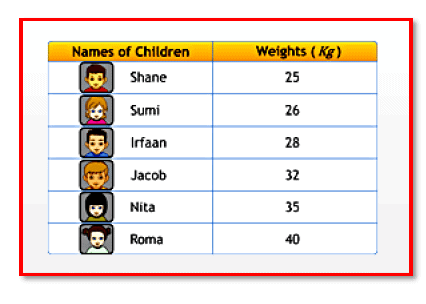Courses

# Chapter Notes - Data Handling Class 7 Notes | EduRev

## Mathematics (Maths) Class 7

Created by: Praveen Kumar

## Class 7 : Chapter Notes - Data Handling Class 7 Notes | EduRev

The document Chapter Notes - Data Handling Class 7 Notes | EduRev is a part of the Class 7 Course Mathematics (Maths) Class 7.
All you need of Class 7 at this link: Class 7

Data RepresentationRepresentation of data using bars of uniform width.
Any information collected can be first arranged in a frequency distribution table, and this information can be put as a visual representation in the form of pictographs or bar graphs. Graphs are a visual representation of organised data.

A bar graph is the representation of data using rectangular bars of uniform width, and with their lengths depending on the frequency and the scale chosen. The bars can be plotted vertically or horizontally. You can look at a bar graph and make deductions about the data.

Bar graphs are used for plotting discrete or discontinuous data, i.e. data that has discrete values and is not continuous. Some examples of discontinuous data are 'shoe size' and 'eye colour', for which you can use a bar chart. On the other hand, examples of continuous data include 'height' and 'weight'. A bar graph is very useful if you are trying to record certain information, whether the data is continuous or not.

Graphs can also be used for comparative analysis.

Double bar graphs are used for comparing data between two different things. The difference between a bar graph and a double bar graph is that a bar graph displays one set of data, and a double bar graph compares two different sets of information or data.

Data ValueArithmetic mean is a number that lies between the highest and the lowest value of data.

Arithmetic mean is a number that lies between the highest and the lowest value of data.

Note -

That we need not arrange the data in ascending or descending order to calculate arithmetic mean.

Range = Highest observation - Lowest observation Mode refers to the observation that occurs most often in a given data.

The following are the steps to calculate mode:
Step - 1: Arrange the data in ascending order.
Step - 2: Tabulate the data in a frequency distribution table.
Step - 3: The most frequently occurring observation will be the mode. Median refers to the value that lies in the middle of the data with half of the observations above it and the other half of the observations below it. The following are the steps to calculate median.

The following are the steps to calculate median:
Step - 1: Arrange the data in ascending order.
Step - 2: The value that lies in the middle such that half of the observations lie above it and the other half below it will be the median. The mean, mode and median are representative values of a group of observations or data, and lie between the minimum and maximum values of the data. They are also called measures of the central tendency.

,

,

,

,

,

,

,

,

,

,

,

,

,

,

,

,

,

,

,

,

,

;